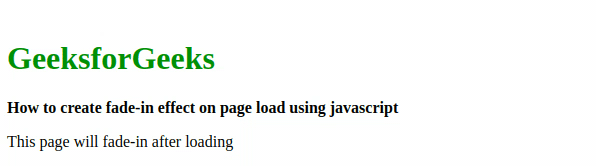# How to add fade-in effect using pure JavaScript?

• Last Updated : 07 May, 2020

The fade effect is described as the gradual increase and decrease in the opacity of the selected portion. In other words, the fade effect is termed as the increase and decrease in opacity with respect to time. When this effect is applied with a gradual increase in the opacity it is known as the fade-in effect. This is the effect that is used to apply fade-in on the selected part on page with the selected interval of time. Fade-out and Fade-in effect is wonderful to use in web projects as it emphasizes on the styling of the web page. We are using setInterval() method and clearInterval() method in these logics. Below we will go with two approaches with the proper description and example.

Using setInterval() method: On page loading, we are calling a function called fadeIn() method. In which we are using setInterval() method which takes two parameters one is function reference(which is the show() in this case) and other is timer(in number), which will call show() function after every given time interval. In show() function we are taking property opacity in variable of name ‘opacity’ and increasing it by 0.1 on every time the fadeIn() function is called. Initially, the opacity of the selected portion is set to 0. Two variables opacity and intervalID is declared inside the script tag. Variable opacity deals with the opacity of the project, while intervalID is used to call clearInterval() function. Then window.onload=fadeIn, is used to call the fadeIn() function automatically. The fadeIn() function calls an in-built method setInterval(), which takes two parameters, one is function reference(show() in this case), and time interval(to take reference of function after selected interval). In setInterval(), show() function is called after every 200 milliseconds in which we are checking opacity of body(variable body holds complete body with the help of id), if it is less than 1 there will be an increment of 0.1 in opacity otherwise clearInterval() function is called which will stop the function calling for show().

Note: The opacity of the selected portion is set to zero to understand the effect.

• Example:

 ` ` `<``html``> ` ` `  `<``head``> ` `    ``<``title``> ` `      ``fade-in effect on page load using JavaScript ` `    `` ` `    ``<``script` `type``=``"text/javascript"``> ` `        ``var opacity = 0; ` `        ``var intervalID = 0; ` `        ``window.onload = fadeIn; ` ` `  `        ``function fadeIn() { ` `            ``setInterval(show, 200); ` `        ``} ` ` `  `        ``function show() { ` `            ``var body = document.getElementById("body"); ` `            ``opacity = Number(window.getComputedStyle(body) ` `                             ``.getPropertyValue("opacity")); ` `            ``if (opacity < ``1``) { ` `                ``opacity` `= opacity + 0.1; ` `                ``body.style.opacity` `= opacity ` `            ``} else { ` `                ``clearInterval(intervalID); ` `            ``} ` `        ``} ` `    `` ` ` ` ` `  `<``body` `id``=``"body"` `style``=``"opacity: 0;"``> ` `    ``<``br``> ` `    ``<``h1` `style``=``"color: green"``>GeeksforGeeks ` `    ``<``b``>  ` `      ``How to create fade-in effect  ` `      ``on page load using javascript  ` `    `` ` `    ``<``p``> ` `      ``This page will fade-in after loading ` `    `` ` ` ` `<``html``> `

• Output:Using clearInterval() method: In this approach, complete logic is written inside a variable which is done with the help of setInterval() method, here complete function is to be written in place of function reference. This approach is comparatively easy to understand. The default value of opacity is 1.So ewe have to set it to zero. to observe the output The window.onload=fadeIn, is used to call the fadeIn() function automatically. Now declaring 3 variables in fadeIn() function:

• fade: It is to fetch the part on which fade-in effect to be applied.
• opacity: It is to deal with the opacity of fetched portion.
• intervalID: It is to deal with complete logic and to terminate logic.

Now instead of writing a function reference, a complete function is to be written, which will be called over and over again after every 200 milliseconds to increase opacity. Now in written function, the opacity of the fetched portion is compared with 1 on each call

• If opacity is found less than 1, the opacity will increase by 0.1 and applied to the fetched portion.
• If opacity is found 1 or greater than 1, clearInterval() function is called to terminate function function calls.
• Example:

 ` ` `<``html``> ` ` `  `<``head``> ` `    ``<``title``> ` `      ``fade-in effect on page load using JavaScript ` `    `` ` `    ``<``script` `type``=``"text/javascript"``> ` `        ``window.onload = fadeIn; ` ` `  `        ``function fadeIn() { ` `            ``var fade = document.getElementById("body"); ` `            ``var opacity = 0; ` `            ``var intervalID = setInterval(function() { ` ` `  `                ``if (opacity < ``1``) { ` `                    ``opacity` `= opacity + 0.1 ` `                    ``fade.style.opacity` `= opacity; ` `                ``} else { ` `                    ``clearInterval(intervalID); ` `                ``} ` `            ``}, 200); ` `        ``} ` `    `` ` ` `  ` ` ` `  `<``body` `id``=``"body"` `style``=``"opacity:0;"``> ` `    ``<``br``> ` `    ``<``h1` `style``=``"color: green"``>GeeksforGeeks ` `    ``<``b``>  ` `      ``How to create fade-in effect  ` `      ``on page load using javascript  ` `    `` ` `    ``<``p``> ` `      ``This page will fade-in after loading ` `    `` ` ` ` ` `  ` `

• Output: This output video is in the loop, that is why it is not stopping after getting opacity 1. It loads again and again automatically.Note: If increment value is greater than 0.1, it fade-in faster. If time interval decreases then also it fade-in faster.

My Personal Notes arrow_drop_up
Recommended Articles
Page :